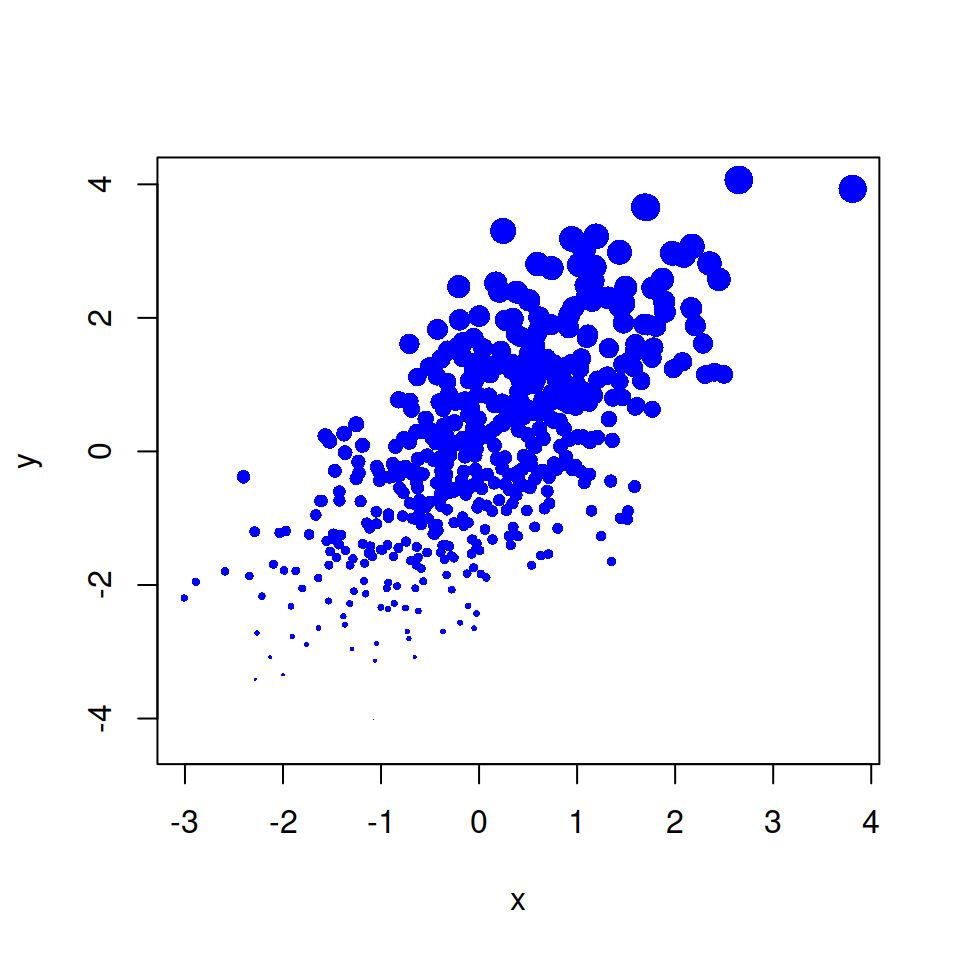# pch symbols in R

Pch stands for ‘plotting character’ and are the symbols used to display the data points on a graph.

## pch symbols list

Most common symbols

The main symbols can be selected with the `pch` argument, with values from 0 to 25.

``````grid <- expand.grid(1:5, 6:1)
plot(grid, pch = 0:30, cex = 2.5,
yaxt = "n", xaxt = "n",
ann = FALSE, xlim = c(0.5, 5.25),
ylim = c(0.5, 6.5))

grid2 <- expand.grid(seq(0.6, 4.6, 1), 6:1)
text(grid2\$Var1[1:26], grid2\$Var2[1:26], 0:25)``````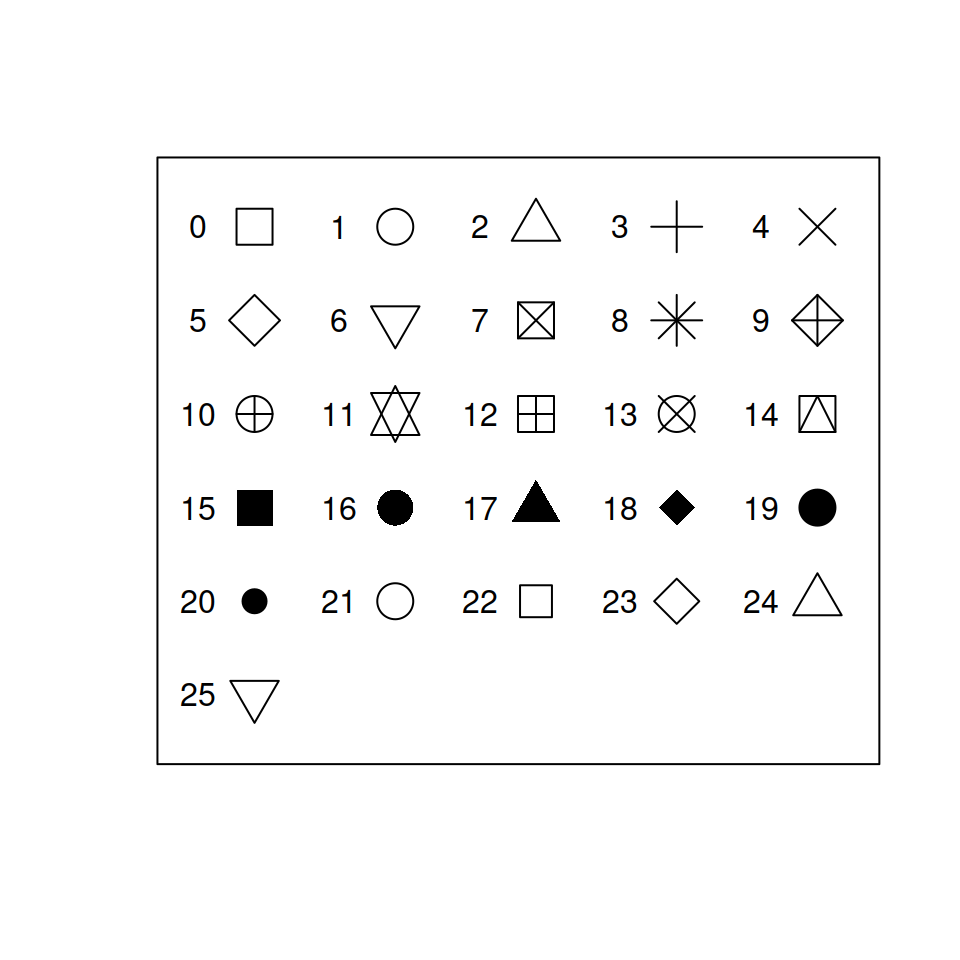Other symbols

The rest can be specified with numbers 32 to 255, which correspond to characters. However, instead of specify its number its easier to set them as a string.

``````# Custom symbols
plot(1:5, 1:5,
pch = c("+", "-", "/", "&", "?"),
cex = 2.5,
xlim = c(0, 6), ylim = c(0, 6),
yaxt = "n", xaxt = "n",
ann = FALSE)``````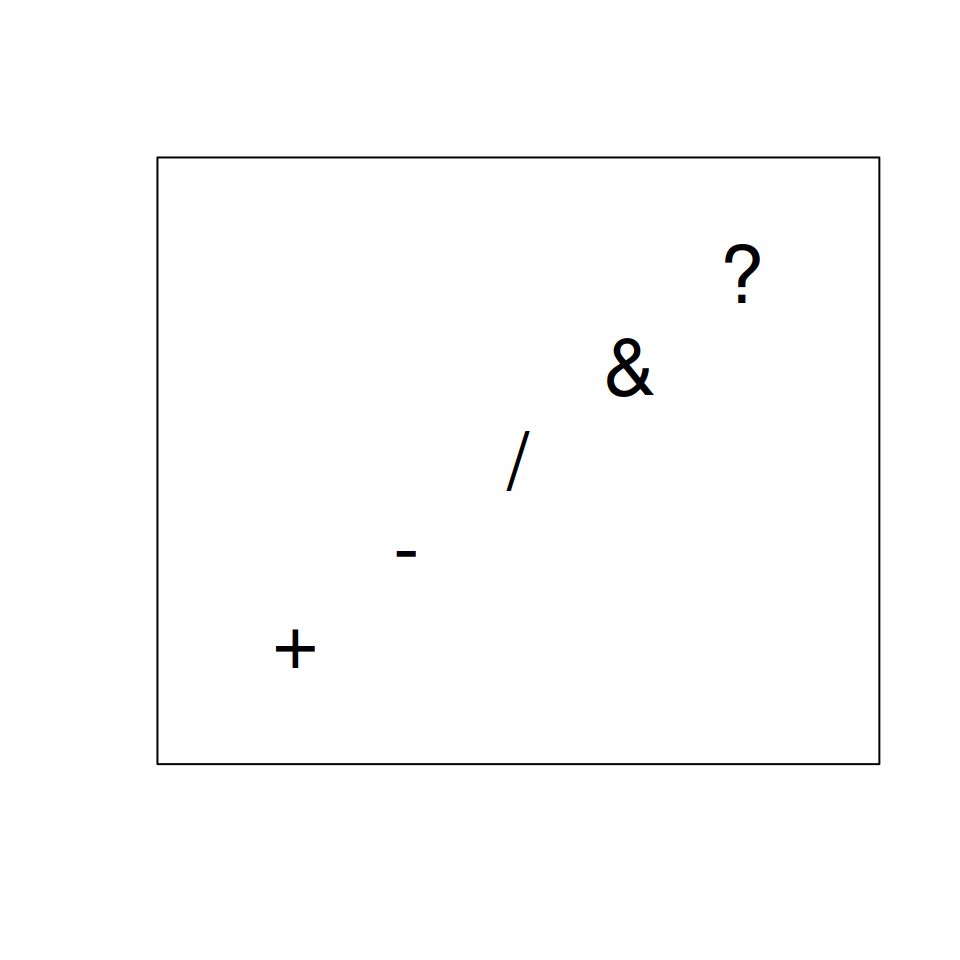The symbols can be classified in several groups, such as:

Symbol Description
0-18 S-compatible vector symbols
19-25 Further R symbols
26-31 Not implemented
32-127 ASCII characters
128-255 Native characters

## pch colors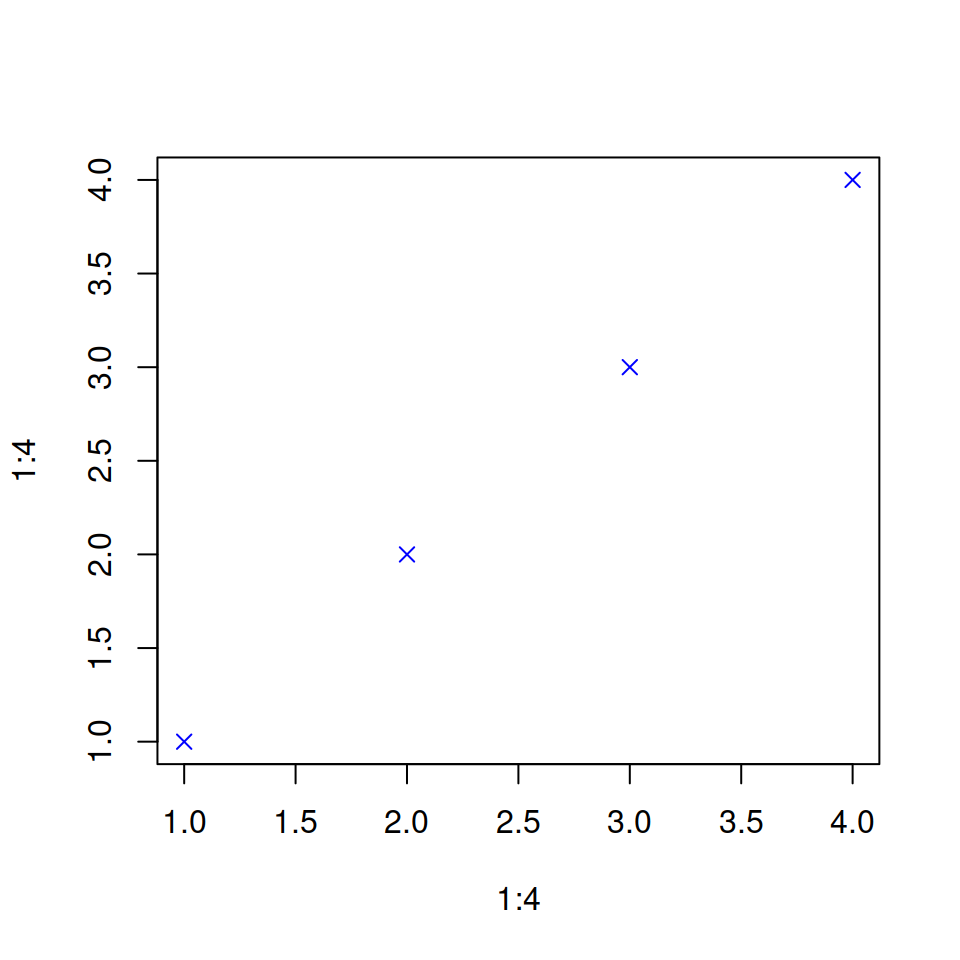Color of the symbols

By default, pch symbols are black, but the color can be changed using `col` argument.

``````plot(1:4, 1:4,     # Data
pch = 4,      # Symbol
col = "blue") # Color``````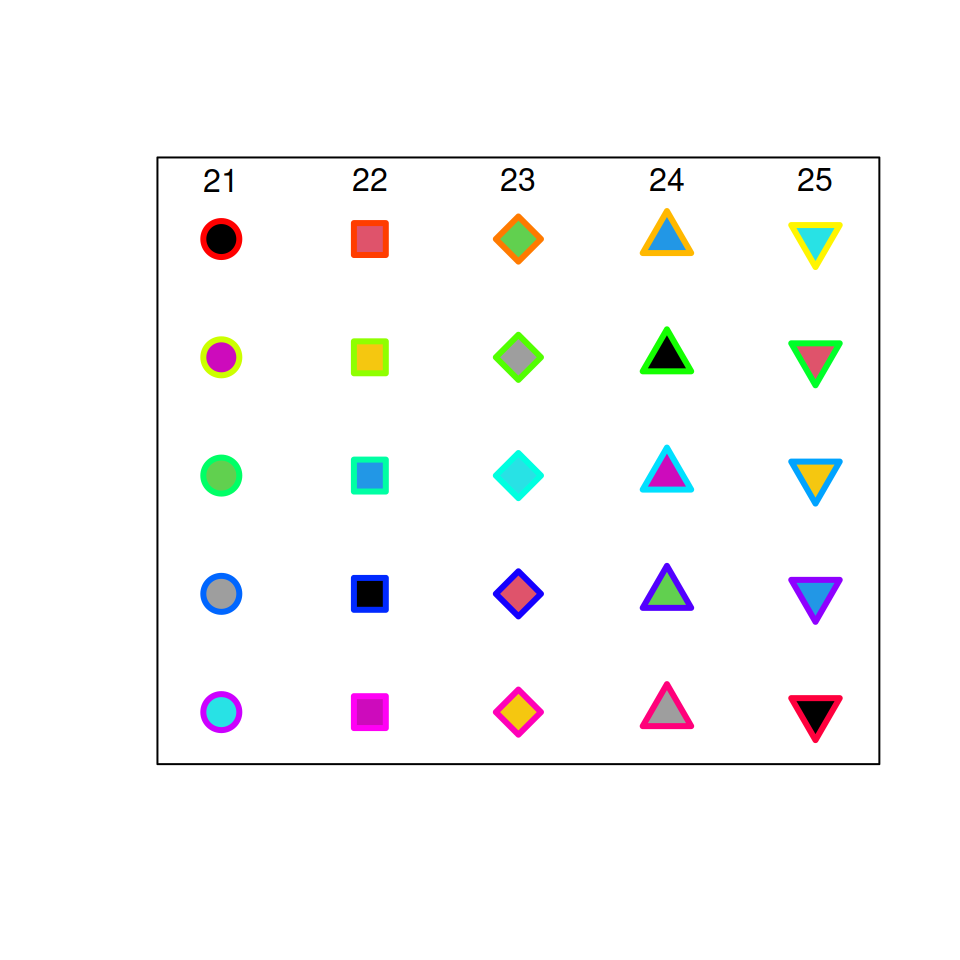Different background color and border color

Symbols 21 to 25 also allow changing the fill color with the `bg` argument and the border color with the `col` argument.

``````grid <- expand.grid(1:5, 5:1)

plot(grid, pch = 21:25, cex = 2.5,
yaxt = "n", xaxt = "n", lwd = 3,
ann = FALSE, xlim = c(0.75, 5.25),
ylim = c(0.75, 5.5),
bg = 1:25, col = rainbow(25))

text(1:5, rep(5.5, 5), 21:25)``````

## pch size

Size of the symbols

You can change the pch size in R with the `cex` argument. Default value is 1.

``````# Generate sample data
set.seed(1)
x <- rnorm(500)
y <- x + rnorm(500)

plot(x, y, pch = 21,
bg = "red",   # Fill color
col = "blue", # Border color
cex = 3,      # Symbol size
lwd = 3)      # Border width``````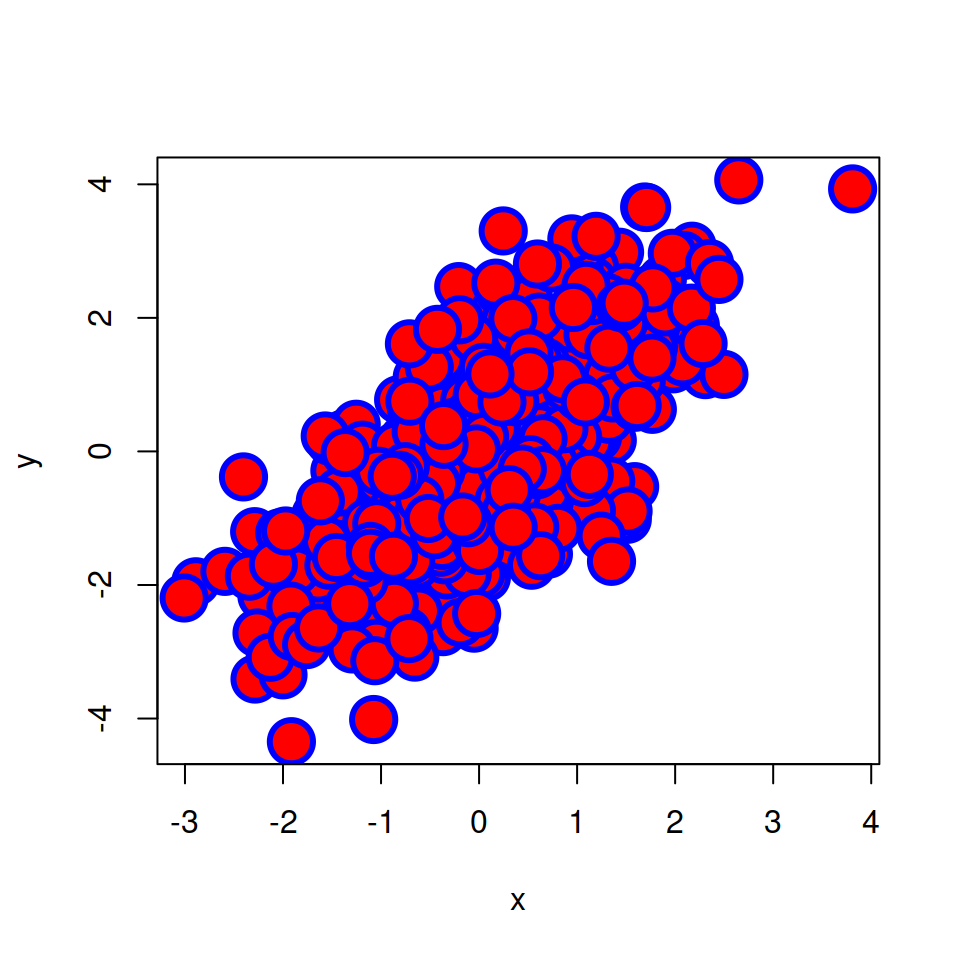Scale the symbols

You can also set the size of the symbol based on a variable, as in the following example.

``````# Generate sample data
set.seed(1)
x <- rnorm(500)
y <- x + rnorm(500)

# Standardize variable Y between 0 and 1
sizes <- (y - min(y))/(max(y) - min(y))

plot(x, y, pch = 16,
col = "blue",    # Color
cex = sizes * 2, # Symbol size
lwd = 3)         # Border width``````